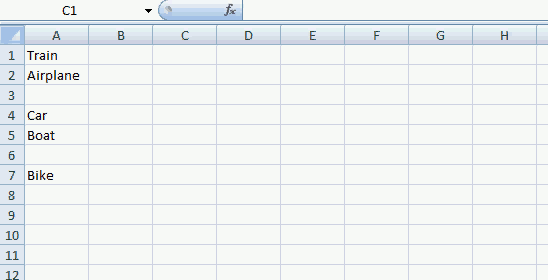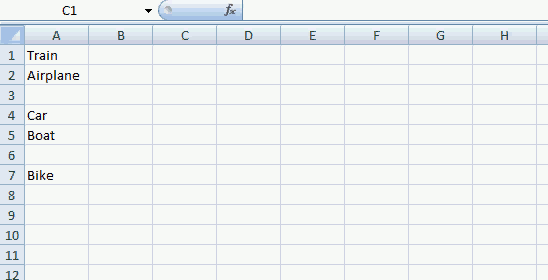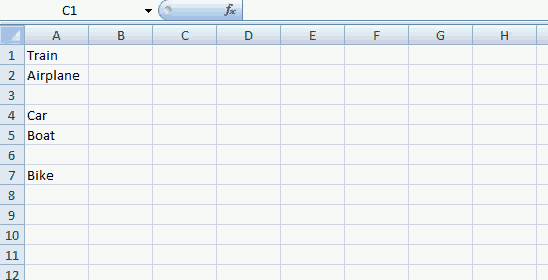Author: Oscar Cronquist Article last updated on August 21, 2019

In this vba tutorial I am going to show you how to return values from an udf, depending on where you enter the udf. All udf examples in this post remove blanks but they differ on how values are returned.

Example 1The values are returned horizontally and #N/A are returned when there are no more values to show.

User defined function

```Function BasicUDF(Rng As Range)
Dim Cell As Variant
'Create an array
Dim temp() As Variant
ReDim temp(0)
'Process every cell in Range
For Each Cell In Rng
'Check if cell is empty
If Cell <> "" Then
'Copy cell value to the last position in array
temp(UBound(temp)) = Cell
'Increase array size with 1
ReDim Preserve temp(UBound(temp) + 1)
End If
Next Cell
'Remove the last value in array
ReDim Preserve temp(UBound(temp) - 1)
'Return values
BasicUDF = temp
End Function
```

You can return values vertically by changing the last line to BasicUDF = Application.Transpose(temp). See animated gif below.Values are transposed vertically.

Example 2

This user defined function returns values vertically and replaces #N/A with blanks.Application.Caller.Rows.Count returns the number of rows from the cell range where you entered the udf. This makes it possible to add blanks when the udf is out of values.

User defined function

```Function BasicUDF2(Rng As Range)
Dim Cell As Variant
Dim row As Single
'Create an array
Dim temp() As Variant
ReDim temp(0)
'Process every cell in Range
For Each Cell In Rng
'Check if cell is empty
If Cell <> "" Then
'Copy cell value to the last position in array
temp(UBound(temp)) = Cell
'Increase array size with 1
ReDim Preserve temp(UBound(temp) + 1)
End If
Next Cell
For row = UBound(temp) To Application.Caller.Rows.Count
temp(UBound(temp)) = ""
ReDim Preserve temp(UBound(temp) + 1)
Next row
'Remove last blank
ReDim Preserve temp(UBound(temp) - 1)
'Transpose temp array from horizontal to vertical and return values to sheet
BasicUDF2 = Application.Transpose(temp)
End Function
```

Example 3

This user defined function returns values in every cell, also if you entered the user defined function in multiple columns. The remaining cells are returned as blanks. See animated gif.Values are transposed to fill the entire selection, #N/A are replaced with blank cells.

User defined function

```Function BasicUDF3(Rng As Range)
Dim Cell As Variant
Dim row As Single, i As Single
'Create an array
Dim temp() As Variant
'Dim array with same size as selection
ReDim temp(Application.Caller.Columns.Count - 1, 0)
i = 0
'Process every cell in Range
For Each Cell In Rng
'Check if cell is empty
If Cell <> "" Then
'Copy cell value to the last position in array
temp(i, UBound(temp, 2)) = Cell
i = i + 1
'Add a new row to the array
If i = Application.Caller.Columns.Count Then
i = 0
ReDim Preserve temp(UBound(temp, 1), UBound(temp, 2) + 1)
End If
End If
Next Cell
'Process remaining cells in selection
Do
'Remaining value are blanks
temp(i, UBound(temp, 2)) = ""
'Count current column
i = i + 1
'Check if current column is equal to the number of columns in selection
If i = Application.Caller.Columns.Count Then
'Start over at column 1 (0)
i = 0
'Add a new row in array
ReDim Preserve temp(UBound(temp, 1), UBound(temp, 2) + 1)
End If
Loop Until UBound(temp, 2) > Application.Caller.Rows.Count - 1
'Remove last row in array
ReDim Preserve temp(UBound(temp, 1), UBound(temp, 2) - 1)
'Return values
BasicUDF3 = Application.Transpose(temp)
End Function
```

How to create an array formula

1. Select a cell range
2. Type user defined function in formula bar
3. Press and hold Ctrl + Shift
4. Press Enter

Where do I copy the vba code?

1. Open VB Editor or press Alt+ F11
2. Press with left mouse button on "Insert" on the menu
3. Press with left mouse button on "Module"
4. Copy/Paste vba code to code module